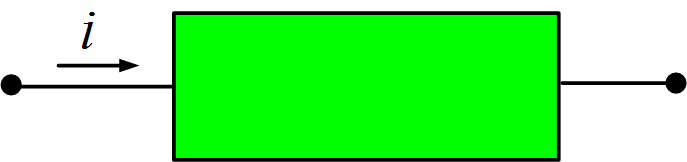# Electric Current | Unit of Electric Current

Want create site? Find Free WordPress Themes and plugins.

The primary purpose of an electric current is to move or transfer charges along specified paths. This motion of charges constitutes an electric current, denoted by the letters i or I, taken from the French word “intensite”. Formally,

Electric CurrentCurrent is the time rate of change of charge, given by

$\begin{matrix} i=\frac{dq}{dt} & \cdots & (1) \\\end{matrix}$

The basic unit of current is the ampere (A), named after Andre-Marie Ampere, a French mathematician, and physicist who formulated laws of electromagnetics. An ampere is 1 coulomb per second.

In circuit theory current is generally thought of as the movement of positive charges. We now know that in metal conductors the current is the movement of electrons that have been pulled loose from the orbits of the atoms of the metal. Thus we should distinguish conventional current (the movement of positive charges), which is used in electric network theory, and electron current.

As an example, suppose the current in the wire of figure 1(a) is I=3A. That is, 3 C/s passes some specified point in the wire. This is symbolized by the arrow labeled 3A, whose direction indicates that the motion is from left to right. This situation is equivalent to that depicted in figure 1(b), which indicated -3C/s or -3A in the direction from right to left.Fig.1: Two representations of the same current

Figure 2 represents a general circuit element with a current i flowing from the left toward the right terminal. The total charge entering the element between time to and t is found by integration. The result is

$\begin{matrix} {{q}_{T}}=q(t)-q({{t}_{o}})=\int\limits_{{{t}_{o}}}^{t}{i\text{ }dt} & \cdots & (2) \\\end{matrix}$

We should note at this point that we are considering the network elements to be electrically neutral. That is, no net positive or negative charge can accumulate in the element. A positive charge entering must be accompanied by an equal positive charge leaving (or, equivalently, an equal negative charge entering). Thus the current shown entering the left terminal in figure 2 must leave the right terminal.Fig.2: Current flowing in a general element

## Electric Current Types

There are several types of current in common use, some of which are shown in figure 3. A constant current, as shown in figure 3 (a), will be termed a direct current, or dc. An alternating current, or AC, is a sinusoidal current, such as that of figure 3(b). Figures 3(c) and (d) illustrate, respectively, an exponential current and a sawtooth current.Fig.3: (a) DC; (b) AC; (c) Exponential Current; (d) Saw-Tooth Current

There are many commercial uses for DC, such as in flashlights and power supplies for electronic circuits, and of course, AC is the common household current found all over the world. Exponential currents appear quite often when a switch is actuated to close the path in an energized circuit. Sawtooth waves are useful in equipment, such as an oscilloscope, used for displaying electrical characteristics on a screen.

## Electric Current Measurement

Current can be measured with a device called an ammeter or clamp meter as shown in figure 4. The ammeter is to be placed in series with the component you are trying to measure to attain a reading.Figure.4: Clamp Meter Working

Typically it is okay to measure small electronic circuits using ammeter where there is a presence of low voltages and current readings. It is not okay, nor is it safe to use this meter or practice this method on residential, commercial, or industrial components or devices due to the fact that your body can act as a conductor and transmit electricity causing bodily harm. In the situation where you need to take current readings in any circuit other than electronics, it is common practice to use a clamp meter or have a device that is built into the equipment needed to be measured.